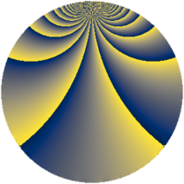# Properties

 Label 378.2.vLevel $378$ Weight $2$ Character orbit 378.v Rep. character $\chi_{378}(67,\cdot)$ Character field $\Q(\zeta_{9})$ Dimension $144$ Newform subspaces $2$ Sturm bound $144$ Trace bound $7$

# Related objects

## Defining parameters

 Level: $$N$$ $$=$$ $$378 = 2 \cdot 3^{3} \cdot 7$$ Weight: $$k$$ $$=$$ $$2$$ Character orbit: $$[\chi]$$ $$=$$ 378.v (of order $$9$$ and degree $$6$$) Character conductor: $$\operatorname{cond}(\chi)$$ $$=$$ $$189$$ Character field: $$\Q(\zeta_{9})$$ Newform subspaces: $$2$$ Sturm bound: $$144$$ Trace bound: $$7$$ Distinguishing $$T_p$$: $$5$$

## Dimensions

The following table gives the dimensions of various subspaces of $$M_{2}(378, [\chi])$$.

Total New Old
Modular forms 456 144 312
Cusp forms 408 144 264
Eisenstein series 48 0 48

## Trace form

 $$144 q + 6 q^{6} + 24 q^{9} + O(q^{10})$$ $$144 q + 6 q^{6} + 24 q^{9} + 24 q^{11} - 6 q^{14} - 12 q^{15} - 24 q^{17} - 30 q^{21} + 42 q^{23} - 36 q^{26} + 6 q^{29} + 18 q^{30} - 36 q^{33} - 36 q^{35} + 6 q^{36} - 72 q^{39} - 12 q^{41} + 48 q^{42} + 6 q^{45} + 18 q^{47} + 36 q^{49} + 12 q^{50} - 36 q^{51} - 30 q^{53} - 36 q^{54} - 6 q^{56} - 6 q^{57} - 60 q^{59} + 18 q^{60} + 18 q^{61} - 48 q^{62} - 60 q^{63} - 72 q^{64} + 54 q^{65} + 18 q^{68} - 60 q^{69} - 18 q^{70} + 24 q^{71} - 12 q^{72} + 72 q^{73} - 36 q^{74} + 12 q^{75} - 30 q^{77} + 36 q^{78} - 36 q^{79} - 12 q^{80} + 48 q^{81} - 6 q^{84} + 72 q^{85} - 24 q^{86} - 156 q^{87} - 72 q^{89} + 36 q^{91} + 42 q^{92} - 96 q^{93} - 36 q^{94} - 30 q^{95} - 24 q^{98} + 36 q^{99} + O(q^{100})$$

## Decomposition of $$S_{2}^{\mathrm{new}}(378, [\chi])$$ into newform subspaces

Label Dim $A$ Field CM Traces $q$-expansion
$a_{2}$ $a_{3}$ $a_{5}$ $a_{7}$
378.2.v.a $72$ $3.018$ None $$0$$ $$0$$ $$0$$ $$-6$$
378.2.v.b $72$ $3.018$ None $$0$$ $$0$$ $$0$$ $$6$$

## Decomposition of $$S_{2}^{\mathrm{old}}(378, [\chi])$$ into lower level spaces

$$S_{2}^{\mathrm{old}}(378, [\chi]) \cong$$ $$S_{2}^{\mathrm{new}}(189, [\chi])$$$$^{\oplus 2}$$